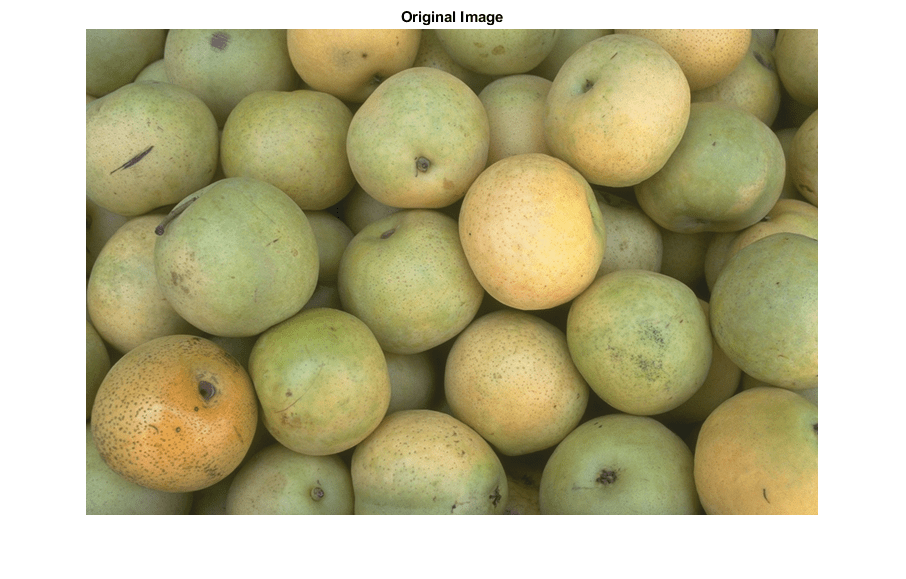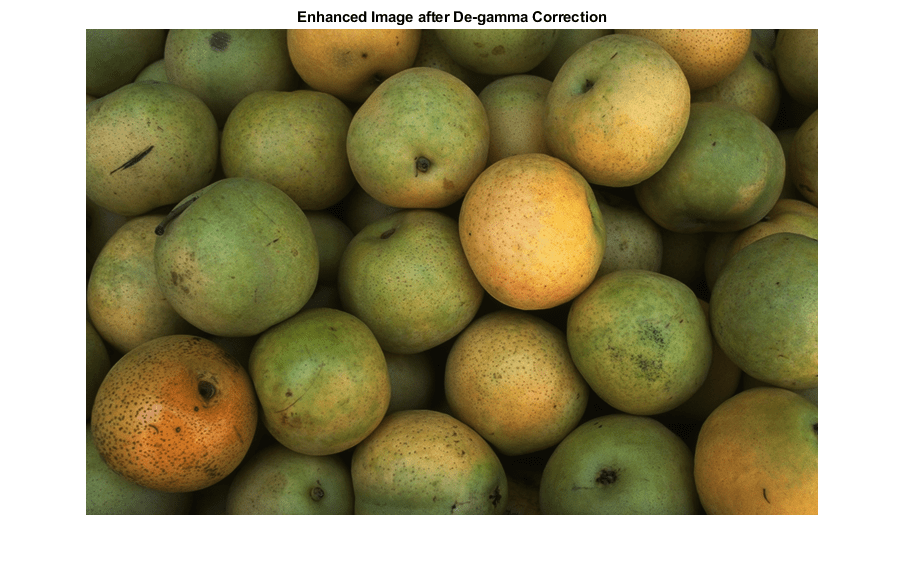# vision.GammaCorrector

Apply or remove gamma correction from images or video streams

## Description

To apply gamma correction to input images or a video stream:

1. Create the `vision.GammaCorrector` object and set its properties.

2. Call the object with arguments, as if it were a function.

## Creation

### Syntax

``gammaCorr = vision.GammaCorrector``
``gammaCorr = vision.GammaCorrector(Name,Value)``
``gammaCorr = vision.GammaCorrector(gamma,Name,Value)``

### Description

example

````gammaCorr = vision.GammaCorrector` returns a gamma corrector object, `gammaCorr`. You can use the gamma corrector to apply or remove gamma correction from images or video streams.```
````gammaCorr = vision.GammaCorrector(Name,Value)` sets properties using one or more name-value pairs. Enclose each property name in quotes. For example, ```gammaCorr = vision.GammaCorrector('Correction','Gamma')``````
````gammaCorr = vision.GammaCorrector(gamma,Name,Value)` additionally sets the `Gamma` property.```

## Properties

expand all

Unless otherwise indicated, properties are nontunable, which means you cannot change their values after calling the object. Objects lock when you call them, and the `release` function unlocks them.

If a property is tunable, you can change its value at any time.

Specify gamma correction or linearization, specified as `'Gamma'` or `'De-gamma'`.

Gamma value of output or input, specified as numeric scalar greater than or equal to `1`. When you set the `Correction` property to `Gamma`, this property gives the desired gamma value of the output video stream. When you set the `Correction` property to `De-gamma`, this property indicates the gamma value of the input video stream.

Enable gamma curve to have linear portion near origin, specified as `true` or `false`.

I-axis value of the end of gamma correction linear segment, specified as a numeric scalar value in the range (0,1). This property applies when you set the `LinearSegment` property to `true`.

## Usage

### Syntax

``y = gammaCorr(input)``

### Description

example

````y = gammaCorr(input)` applies or removes gamma correction from input `I`, and returns the gamma corrected or linearized output `y`.```

### Input Arguments

expand all

Input, specified as an M-by-N matrix of intensity values or M-by-N-P color video, where P is the number of color planes.

### Output Arguments

expand all

Output, returned as an M-by-N matrix of intensity values or M-by-N-P color video, where P is the number of color planes.

## Object Functions

To use an object function, specify the System object™ as the first input argument. For example, to release system resources of a System object named `obj`, use this syntax:

`release(obj)`

expand all

 `step` Run System object algorithm `release` Release resources and allow changes to System object property values and input characteristics `reset` Reset internal states of System object

## Examples

collapse all

Create a gamma corrector object with the gamma property set to `2.0.`

`hgamma = vision.GammaCorrector(2.0,'Correction','De-gamma');`

`img = imread('pears.png');`

Apply gamma correction.

`imgCor = hgamma(img);`

Show the original and corrected images.

`imshow(img); title('Original Image');````figure, imshow(imgCor); title('Enhanced Image after De-gamma Correction');```